﻿ Lateral Rotor Vibration Analysis Model

### Lateral Rotor Vibration Analysis Model

Robert Surovec, Jozef Bocko, Juraj ŠarlošiOPEN ACCESSPEER-REVIEWED

## Lateral Rotor Vibration Analysis Model

Robert Surovec1, Jozef Bocko2, Juraj Šarloši1,1ZTS VVÚ a s. Južná trieda 95, 041 24 Košice, Slovakia

2Faculty of Mechanical Engineering Technical University of Kosice Letná 9, 042 00 Košice, Slovakia

### Abstract

In this article we examine the lateral vibrations in rotating machinery can lead to rotor unstable behaviour. In some types of rotating machinery, the angular speed is subjected to wide ranges of change. The problem is to find the range of angular speeds for which a machine exhibits stable behaviour in the presence of the coupling of dynamical modes. For determine of mathematical model for vibration we will use Föppl-Jeffcott rotor model. We will examine a undamped and damped vibration on the Föppl-Jeffcott rotor model.

### At a glance: Figures

1
Prev Next

• Surovec, Robert, Jozef Bocko, and Juraj Šarloši. "Lateral Rotor Vibration Analysis Model." American Journal of Mechanical Engineering 2.7 (2014): 282-285.
• Surovec, R. , Bocko, J. , & Šarloši, J. (2014). Lateral Rotor Vibration Analysis Model. American Journal of Mechanical Engineering, 2(7), 282-285.
• Surovec, Robert, Jozef Bocko, and Juraj Šarloši. "Lateral Rotor Vibration Analysis Model." American Journal of Mechanical Engineering 2, no. 7 (2014): 282-285.

 Import into BibTeX Import into EndNote Import into RefMan Import into RefWorks

### 1. Introduction

In this paper transient and steady-state coupled lateral torsional vibrations of the rotating machine were investigated by means of the discrete-continuous mechanical model. For this purpose we use Föppl/Jeffcott single mass rotor. Relatively simple geometrical shape of this real rotor shaft with rigid attached rotor enable us to create a mathematical model for undamped free vibration and damped free vibration. We also determine natural frequency for both case

### 2. Introduction to Rotor Dynamics

Rotor dynamics is the branch of engineering that studies the lateral and torsional vibrations of rotating shaft, with the objective of predictive the rotor vibrations and containing the vibration level under an acceptable limit. Lateral rotor vibration (LRV) is radial-plane orbital motion of the rotor spin axis. Transverse rotor vibration is used synonymously for LRV. Actual LRV orbits are typical only a few hundredths of a millimeter. LRV is an important design consideration in many types of rotating machinery, particularly turboelectrical machines such as steam and combustion gas turbine/generator sets, compressors, pump, gas turbine jet engines, turbochargers and electric motors. Thus LRV affect several major industries. Usually, but not always, the potential for rotor dynamic beam-bending-type deflection significantly contributes to the LRV characteristics. The significance of LRV rotor bending increases with bearing-to-rotor stiffness ratio and with rotor spin speed. Consequently, in some rotating machines with a low operating speed and /or low bearing-to-rotor stiffness ratio, the LRV is essentially of a rigid rotor vibrating in flexible bearing or support. The opposite case (a flexible rotor in essentially rigid bearings) is also possible but rotor dynamically less desirable, because it lacks some vibratory motion at the bearings which often provides that essential ingredients, damping, to keep vibration amplitudes at resonance conditions within tolerable levels. A rotor’s flexibility and mass distributions and it is bearings flexibilities combined with its maximum spin speed essentially determine whether or not residual rotor unbalance can produce forced LRV resonance. That is these factors determine whether the rotor-bearing system has one or more lateral natural frequency modes below the operating speed. If so, then the rotor must pass through the speed (critical speed) where the residual mass unbalances act as once-per-revolution harmonic forces to excite the one or more natural frequencies the rotor speed traverses when accelerating to operating speed and when coasting down. Resonate mode shapes at critical speeds are also determined by the same rotor and bearing properties. Many types of modern rotating machinery are designed to operate above one or more critical speed because of demand for compact high-performance machines. When one or more speed are to be traversed, LRV analyses are required at the design stage of a rotating machine. These analyses generally include computations to ensure that the machine is not inadvertently designed to run continuously at or near a critical speed. These analyses should also include computed unbalance rotor vibration levels over the entire range to ensure that the rotor-bearing system is adequately damped to pass safely through the critical speeds within the operating speed range.

2.1. Föppl/Jeffcott Single Mass Rotor

The vibration theory for rotor-dynamics system was first developed by August Föppl in 1895 and Henry Homan Jeffcot in 1919. Employing a simplified rotor/bearing systems they developed the basic theory on prediction and attenuation of rotor vibration. This simplified rotor/bearing system that is commonly known as the Föppl/Jeffcot rotor, or simply Jeffcot rotor, is often employed to evaluate more complex rotor-dynamic systems in the real world. The rotor disk mass m is located at the axial center of the shaft. The mass of the shaft in the Jeffcot rotor is assumed to be negligible compared to that of the disk, and thus is considered to be massless during the analysis. The geometric center of the disk C is located at the point (uxC, uyC) along coordinate axis defined about the bearing center line, and the disk center mass G is located at (uxG, uyG). The unbalance eccentricity eu is the vector connecting the point C and G, and it represents the unbalance in the rotor disc. The rotating speed of the disc/shaft is given by ω, and without loss of generality we assume that eu is parallel with the x-axis at the initial time t = 0 .

Under the assumption that the rotor disk does not affect the stiffness of the massless shaft, the lateral bending stiffness at the axial center of a simply supported uniform beam is given by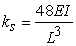(1)

Where E is the elastic modulus of the beam, L is the length between the bearings, I is the shaft area moment of inertia, and D is diameter of the cylindrical shaft.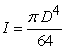(2)

Additionally, we assume that there is a relatively small effective damping action on the lateral motion of the disk at the rotor midspan, and the corresponding damping constant is given by cs. The dynamic equations for the Föppl/Jeffcott rotor are derived by applying Newton´s law to the rotor disk. With the assumption that the shaft is massless, the force acting on the disk are the inertial force and the stiffness/damping force generated by the lateral deformation of the shaft. The lateral equations of the motion in the x – and – y – axes as show in figure 1 are found to be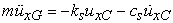(3)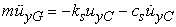(4)

Where (uxC, uyC) and (uxG, uyG) are the coordinates of the geometric center and mass center, respectively. The coordinates of the disk center of mass can be rewritten in terms of its geometric center C and the rotor angle of rotation ωt at time t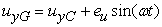(5)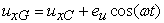(6)

Substituting the second time derivative of previous equations we obtain the equations of motion for the Föppl/Jeffcott rotor in terms of the disk geometric center as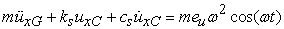(7)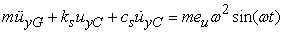(8)

We note here that, as the bearings are considered to be infinitely stiff and the rotor disk does not tilt, this model does not include the gyroscopic effects acting on the rotor.

2.2. Damped Free Vibration

We consider the free vibration of the Föppl/Jeffcott rotor with a non-zero effective shaft damping action on the system. Newton´s equations of motion in eqs.(7),(8) becomes.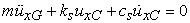(9)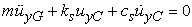(10)

The solutions to the above systems of homogeneous second order differential equations take the form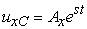(11)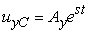(12)

Substituting these solutions into (11), (12) we obtain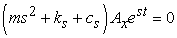(13)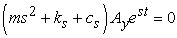(14)

These equations hold for any initial condition if the damped characteristic equation holds: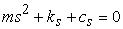(15)

The zeros of the characteristic equation, also know as the damped eigenvalues of the system, are found to be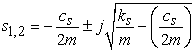(16)

Generally, the rotor/bearing system is underdamped, which means that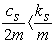(17)

And s will have an imaginary component. Define the damping ratio as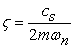(18)

This value corresponds to the ratio of the effective damping cs to the critical value in the damping constant when the system become over damped, or the imaginary part of the solution in Eq.(16) vanishes. Eqs. (13)and(14) can be rewritten as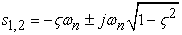(19)

The imaginary component of s is know as the damped natural frequency,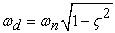(20)

The damping coefficient 0.1 is normally consider as needed for the safe operation of the machine. The final solutions to the undamped free vibration are found to be the linear combination of the (11,12) and (16), that is,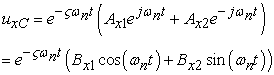(21)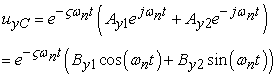(22)

For some values of Axi and Ayi, or Bxi and Byi, dependent on the initial condition of the rotor .

2.3. Undamped Free Vibration

The undamped tree vibration analysis deals with rotor vibration in the case of negligible unbalance eccentricity (eu = 0) and damping (cs = 0). The equations of motion in (7),(8) are simplified to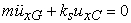(23)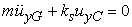(24)

The solution to this second order homogenous system takes the form: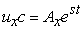(25)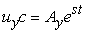(26)

For some complex constant s. The values of the constant Ax and Ay are obtained from the initial condition of the rotor disk. Substituting the solution in Eqs. (25),(26) into Eqs. (23),(24) we obtain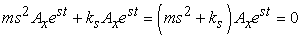(27)(28)

The above equations hold true for any value of Ax and Ay if the undamped characteristic equation holds,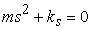(29)

Solving the above equality for the complex constant s, we obtain the following solution: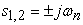(30)

Where ωn is the undamped natural frequency of the shaft defined as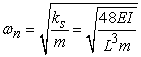(31)

The undamped critical speed of the system is defined as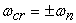(32)

corresponding to the positive + ωD and the negative backward - ωD components. The forward component indicate the lateral vibration that follows the direction of the shaft rotation, and the backward component represent the vibration that moves in the opposite direction. The final solutions to the undamped free vibration are given by the linear combination of the two solutions found in Eqs. (33), (32) ,(33)

And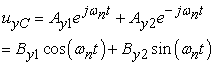(34)

For some values of Axi and Ayi, or Bxi and Byi, which can be found from the initial conditions of the rotor.

We observe that the response is oscillatory, where the frequency is given by the damped natural frequency ωd. Because of the damping, the magnitude of the oscillator is reduced over time, and the rate of decays is a function of the damping ratio ζ and the undamped natural frequency ωn.

### Acknowledgement

The authors gratefully acknowledge support from Slovak Grant Agency VEGA – grant VEGA 1/1205/12 Numerical modeling of mechatronic systems.

The research presented in this paper was also supported by Competence Center for Innovation Knowledge Technology of production systems in industry and services (ITMS project code 26220220155).

### References

  W.J. Palm, Mechanical Vibrations, J. Waley 2007.In article  A.A.Shabana, Theory of Vibrations, Sprinder Science and Busines Media, 8.12.1995.In article  W.Thpmpson, Theory of Vibrations with Application,CRC Press,1.2.1996.In article  Kelly, Mechanical Vibrations, MC Graw – Hill Education India Pvt Limited 1962.In article  F.Robert,Steider Jr., An Introduction to Mechanical Vibrations, paper back – January 17.1989.In article  B.Srikant, Mechanical Vibrations, Person Education India 2010.In article PubMed